# Ncert Class 6 Math Fractions Exercise 7.1

Fractions Class 6 Ex. 7.1

New Ncert Class 6, Chapter 7 Math Free Solution.

Exercise 7.1

Question 1 :- Write the fraction representing-shaded portion.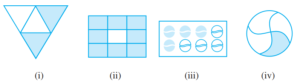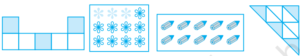(v)                        (vi)                    (vii)                    (viii)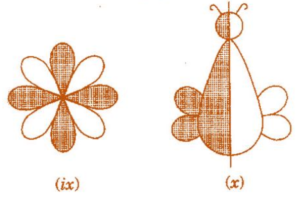Solution 1:-
(i) Total number of parts = 4
Number of shaded parts = 2
∴ Fraction = 2/4

(ii) Total number of parts = 9
Number of shaded parts = 8
∴ Fraction = 8/9

(iii) Total number of parts = 8
Number of shaded parts = 4
∴ Fraction = 4/8

(iv) Total number of parts = 4
Number of shaded parts = 1
∴ Fraction = 1/4

(v) Total number of parts = 7
Number of shaded parts = 3
∴ Fraction = 3/7

(vi) Total number of parts = 12
Number of shaded parts = 3
∴ Fraction = 3/12

(vii) Total number of parts = 10
Number of shaded parts = 10
∴ Fraction = 10/10

(viii) Total number of parts = 9
Number of shaded parts = 4
∴ Fraction = 4/9

(ix) Total number of parts = 8
Number of shaded parts = 4
∴ Fraction = 4/8

(x) Total number of parts = 2
Number of shaded part = 1
∴ Fraction = 1/2

Question 2 :- Colour the part according to the given fraction.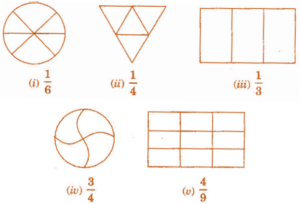Solution 2:-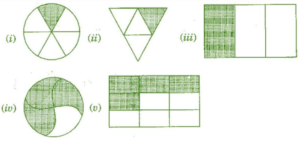Question 3 :- Identify the error, if any.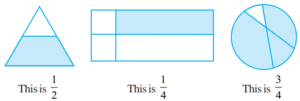Solution 3:-
(a) due to the shaded portion not being half.
∴ This is not 1/2.

(b) Since, the parts are not equal.
∴ Shaded part is not 1/4 .

(c) Since, the part are not equal.
∴ Shaded part is not 3/4.

All the figures are not equally divided. For making fractions, it is necessary that figure is to be divided in equal parts.

Question 4 :- What fraction; of a day is 8-hours?
Solution 4:-
Since we have 8 hours every day and a day has 24 hours,
∴ Required fraction = 8/24.

Question 5 :- What fraction; of a-hour is 40-minutes?
Solution 5:-
Since I hours = 60 minutes
∴ Fraction of 40 minutes = 40/60

Question 6 :- Arya, Abhimanyu and Vivek; shared lunch. Arya has brought two-sandwiches, one made of vegetable and one of Jam. The other two-boys forgot to bring their lunch. Arya agreed to share his sandwiches so that each person will have an equal(=) share of each-sandwich.
(a) How can Arya divide his-sandwiches so that each-person has an equal-share?
(b) What part; of a sandwich will each-boy receive?

Solution 6:-
(a) In order to make three equal portions, Arya divided his sandwich.
They will each receive one part as a result.
(b) They will each get a 1/3 of the total.
∴ Required fraction = 1/3

Question 7 :- Kanchan dyes dresses. She had to dye 30 dresses. She has so far finished 20 dresses. What fraction of dresses has she finished?
Solution 7:-
Total number of dresses which to be dyed = 30
Number of dresses finished = 20
∴ Required fraction = 20/30 = 2/3

Question 8 :- Write the natural-numbers; from 2 to 12. What fraction of them are prime numbers?
Solution 8:-
Natural numbers between 2 and 12 are;
2, 3, 4, 5, 6, 7, 8, 9, 10, 11, 12
Number of given natural numbers = 11
Number of prime numbers = 5
∴ Required fraction = 5/11

Question 9 :- Write the natural-numbers, from 102 to 113. What fraction of them are prime numbers?
Solution 9:-
Natural numbers from 102 to 113 are;
102,103,104,105,106, 107,108, 109,110, 111, 112,113
Total number of given natural numbers = 12
Prime numbers are 103, 107, 109, 113
∴ Number of prime numbers = 4
∴ Required fraction = 4/12 = 1/3

Question 10 :- What fraction; of these circle have X’s in them?Solution 10:-
Total number’of circles = 8
4 is the number of circles with Xs in them.
Required fraction = 4/8 = 1/2

Question 11 :- Kristin, received a CD-player for her birthday. She bought 3-CDs and received 5-others as gifts. What fraction; of her total CDs did she buy and what fraction; did she receive as gifts?
Solution 11:-
Number of CDs bought by her from the market = 3